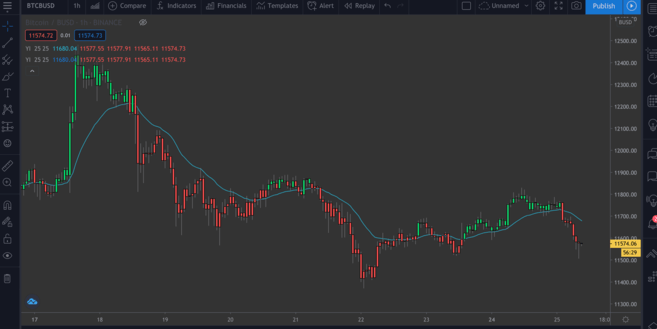# 如何使用 TradingView 建立 TA 指標

13m

## 什麼是 Pine Script？

Pine Script 是一種腳本語言，可用來修改您的 TradingView 圖表。雖然平台已經提供了許多功能，但 Pine Script 可以讓您更進階使用。無論您想改變 K 線圖的顏色，或是想回測新的策略，Pine 編輯器都能讓您依照您的需求，自訂您的即時圖表。

## 設定

Pine Script 非常容易上手。我們所編寫的任何程式碼都在 TradingView 伺服器上執行，因此可以在瀏覽器上使用編輯器來開發我們的腳本 – 不需要另外下載或進行額外的設定。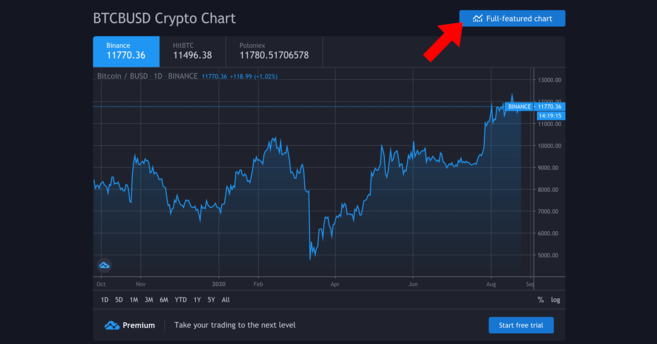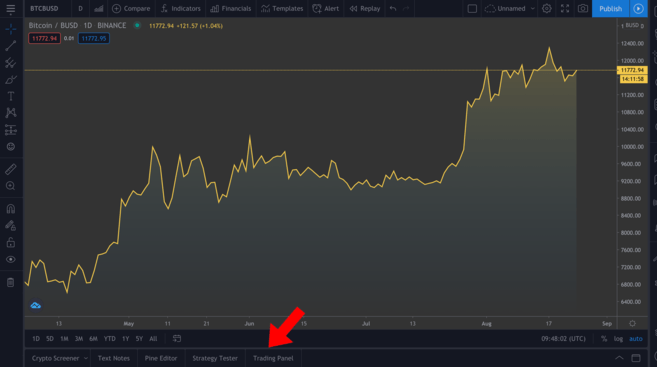## Pine 編輯器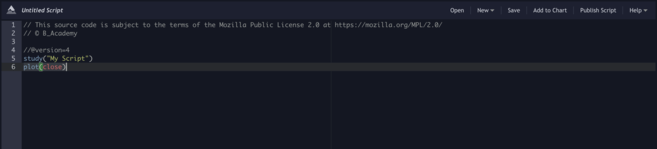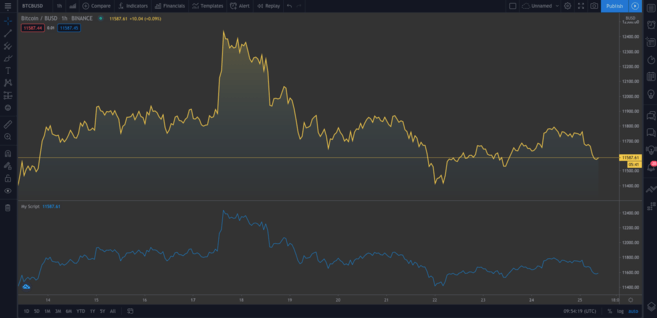`study("My Script")`

`plot(close)`

`//@version=4 study("My Script", overlay=true) plot(open, color=color.purple)`

`//@version=4 study("My Script", overlay=true) plotcandle(open, high, low, close)`

`//@version=4 study("My Script", overlay=true) colors = open >= close ?color.red : color.green plotcandle(open, high, low, close)`

`//@version=4 study("My Script", overlay=true) colors = open >= close ?color.red : color.green plotcandle(open, high, low, close, color=colors)`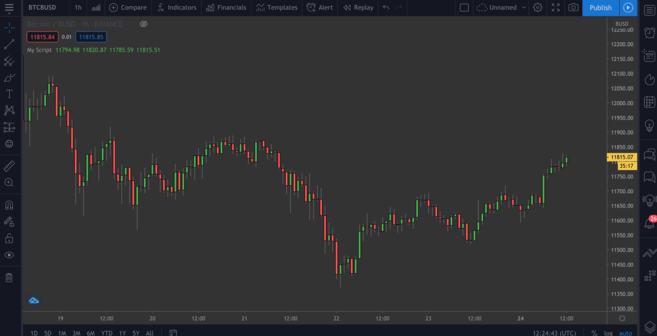## 繪製移動平均線 (MA)

EMA 與簡單移動平均線 (SMA) 略有不同，因為它更重視最新的資料。它對突發的波動更加敏感，通常用於短期交易（例如，日間交易）。

### 簡單移動平均線 (SMA)

`plot(sma(close, 10))`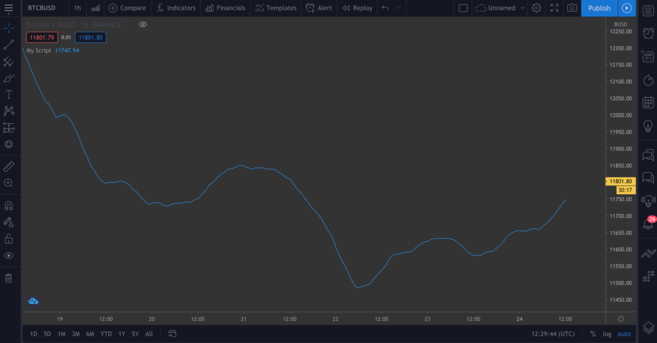### 指數移動平均線 (EMA)

EMA 會有點難以理解，但是不用擔心。我們先來分解這個公式：

`EMA = (Close - Previous Day’s EMA) * Multiplier - Previous Day’s EMA`

`Multiplier = 2 / (Length of EMA + 1)`

`//@version=4 study("My Script", overlay=true) plot(sma(close, 10)) plot(ema(close,10))`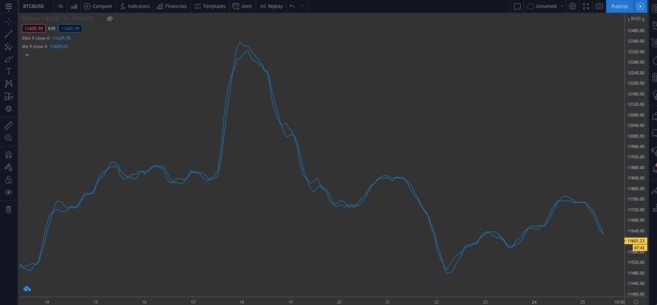### 內建腳本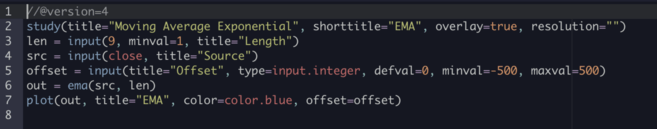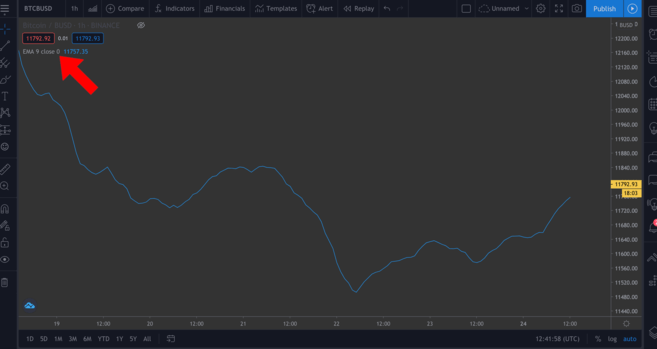...按一下設定齒輪圖示，即可輕鬆變更彈出視窗中的一些值。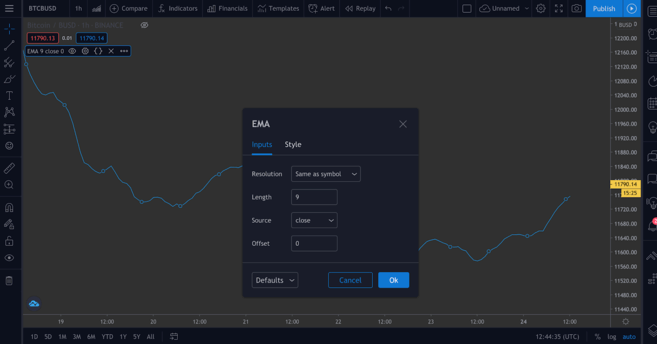## 回測

`//@version=4 strategy("ToDaMoon", overlay=true) enter = input(11000) exit = input(11300) price = close if (price <= enter)     strategy.entry("BuyTheDip",strategy.long,comment="BuyTheDip") if (price >= exit)     strategy.close_all(comment="SellTheNews")`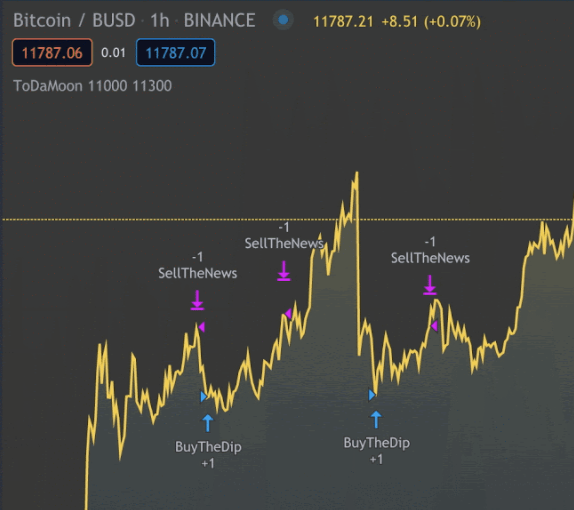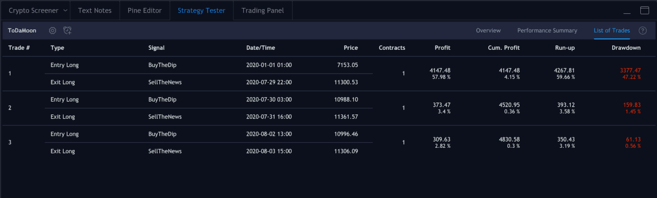## 整合在一起

`study(title="Binance Academy Script", overlay=true)`

`study(title="Binance Academy Script", overlay=true) emaLength = input(title="EMA Length", type=input.integer,defval=25, minval=0)`

`study(title="Binance Academy Script", overlay=true) emaLength = input(title="EMA Length", type=input.integer,defval=25, minval=0) emaVal = ema(close, emaLength)`

`study(title="Binance Academy Script", overlay=true) emaLength = input(title="EMA Length", type=input.integer,defval=25, minval=0) emaVal = ema(close, emaLength) rsiLength = input(title="RSI Length", type=input.integer,defval=25, minval=0)`

`study(title="Binance Academy Script", overlay=true) emaLength = input(title="EMA Length", type=input.integer,defval=25, minval=0) emaVal = ema(close, emaLength) rsiLength = input(title="RSI Length", type=input.integer,defval=25, minval=0) rsiVal = rsi(close, rsiLength)`

`study(title="Binance Academy Script", overlay=true) emaLength = input(title="EMA Length", type=input.integer,defval=25, minval=0) emaVal = ema(close, emaLength) rsiLength = input(title="RSI Length", type=input.integer,defval=25, minval=0) rsiVal = rsi(close, rsiLength) colors = close > emaVal and rsiVal > 50 ?color.green : color.red`

`study(title="Binance Academy Script", overlay=true) emaLength = input(title="EMA Length", type=input.integer,defval=25, minval=0) emaVal = ema(close, emaLength) rsiLength = input(title="RSI Length", type=input.integer,defval=25, minval=0) rsiVal = rsi(close, rsiLength) colors = close > emaVal and rsiVal > 50 ?color.green : color.red plot(emaVal, "EMA")`

`study(title="Binance Academy Script", overlay=true) emaLength = input(title="EMA Length", type=input.integer,defval=25, minval=0) emaVal = ema(close, emaLength) rsiLength = input(title="RSI Length", type=input.integer,defval=25, minval=0) rsiVal = rsi(close, rsiLength) colors = close > emaVal and rsiVal > 50 ?color.green : color.red plot(emaVal, "EMA") plotcandle(open, high, low, close, color=colors)`